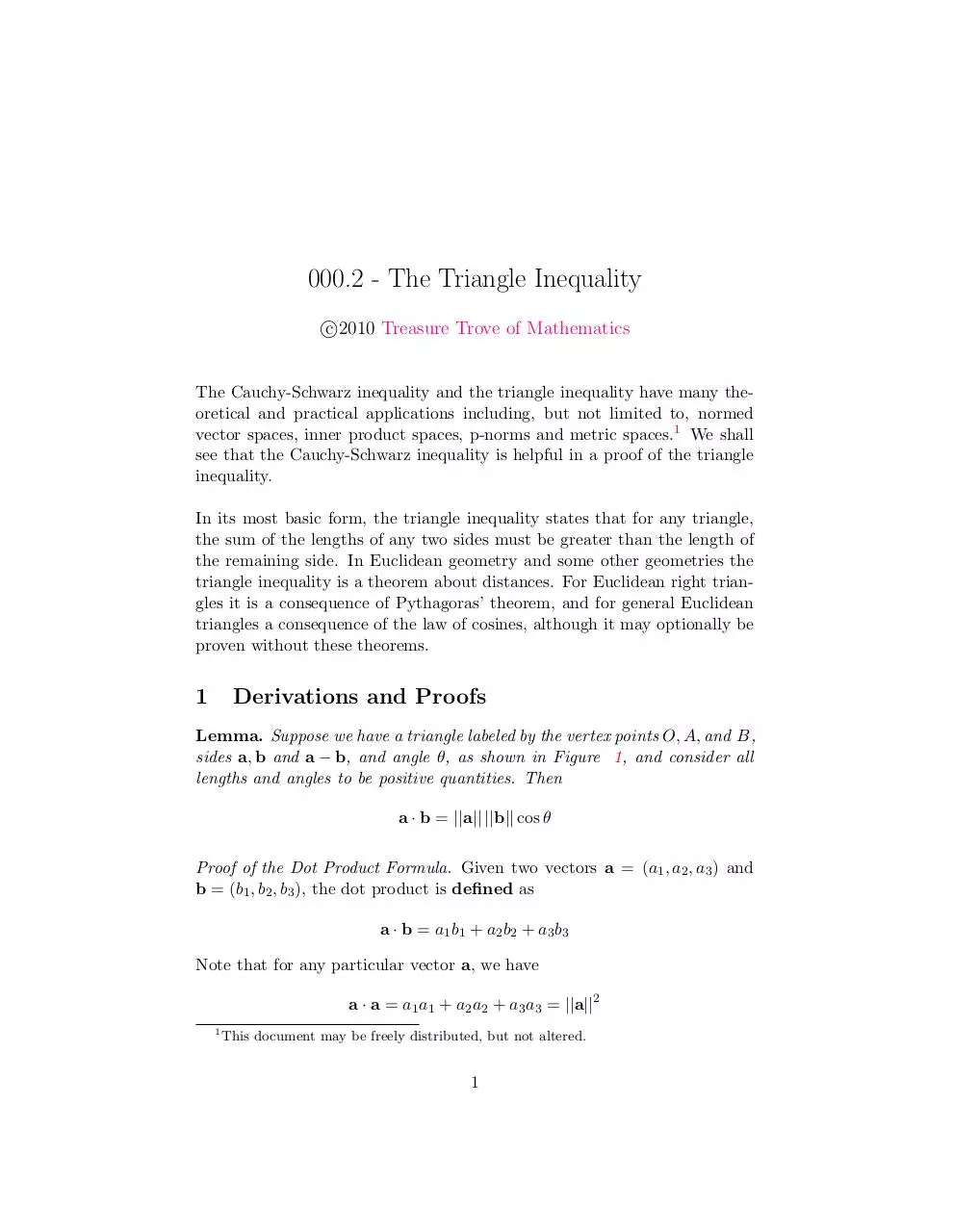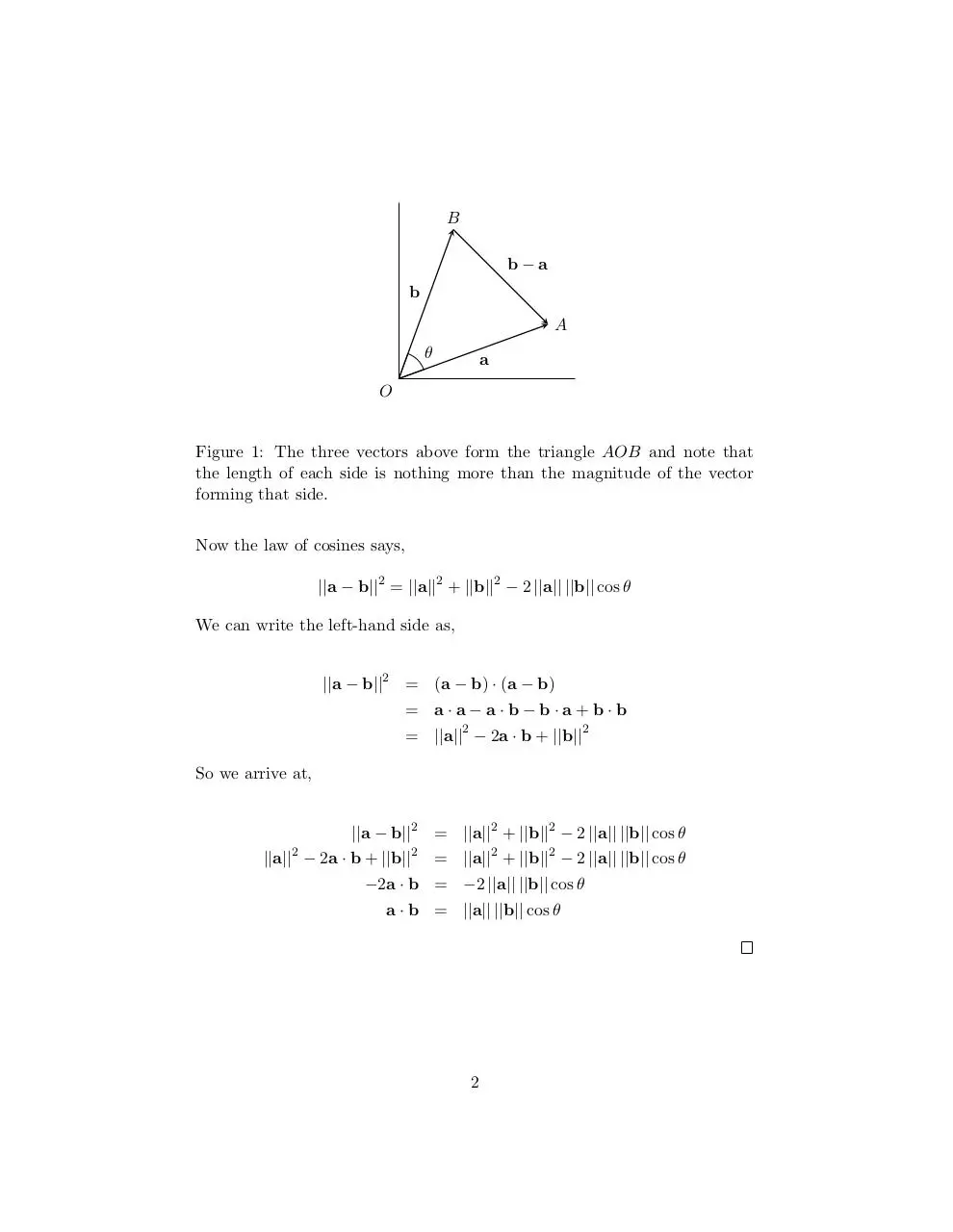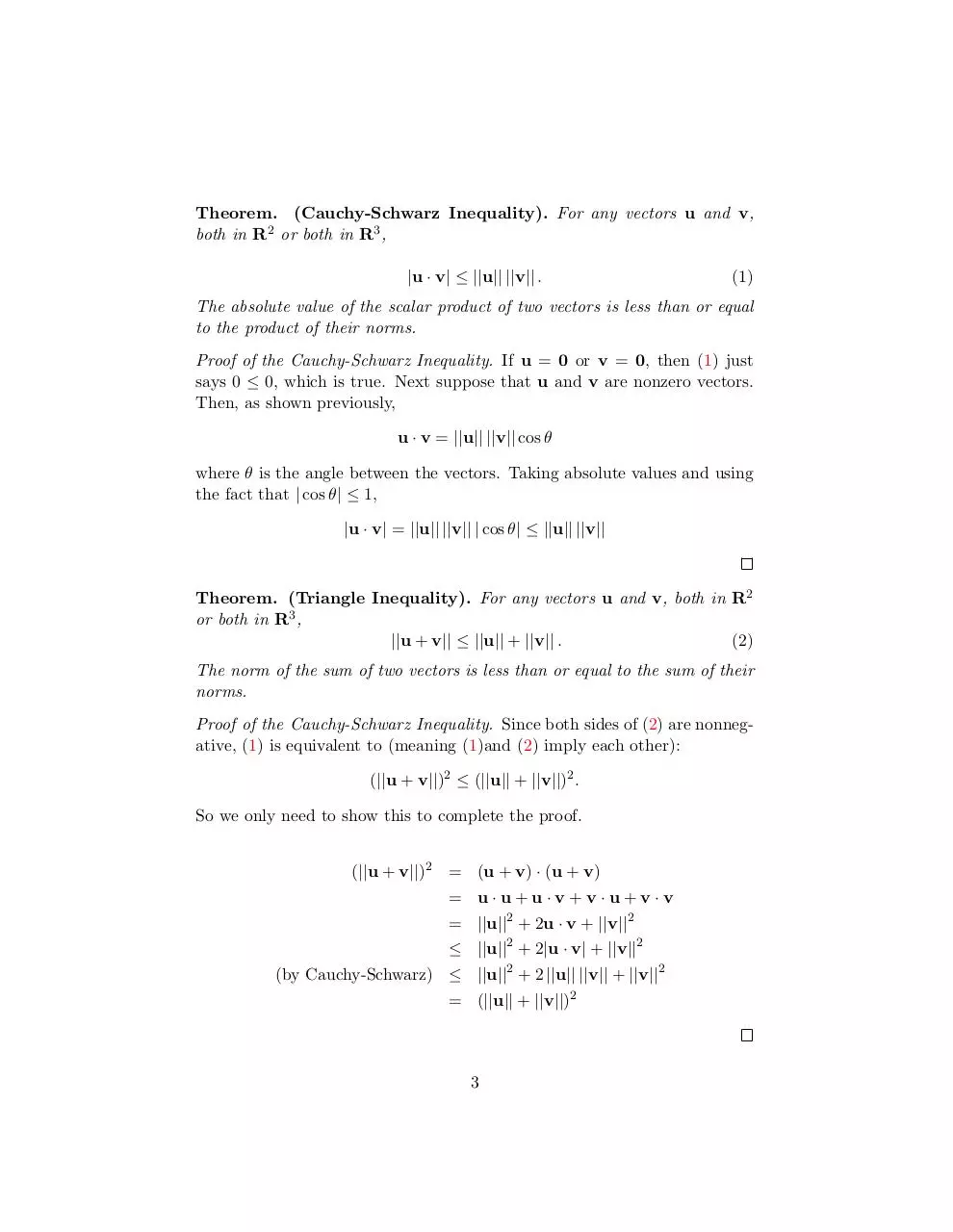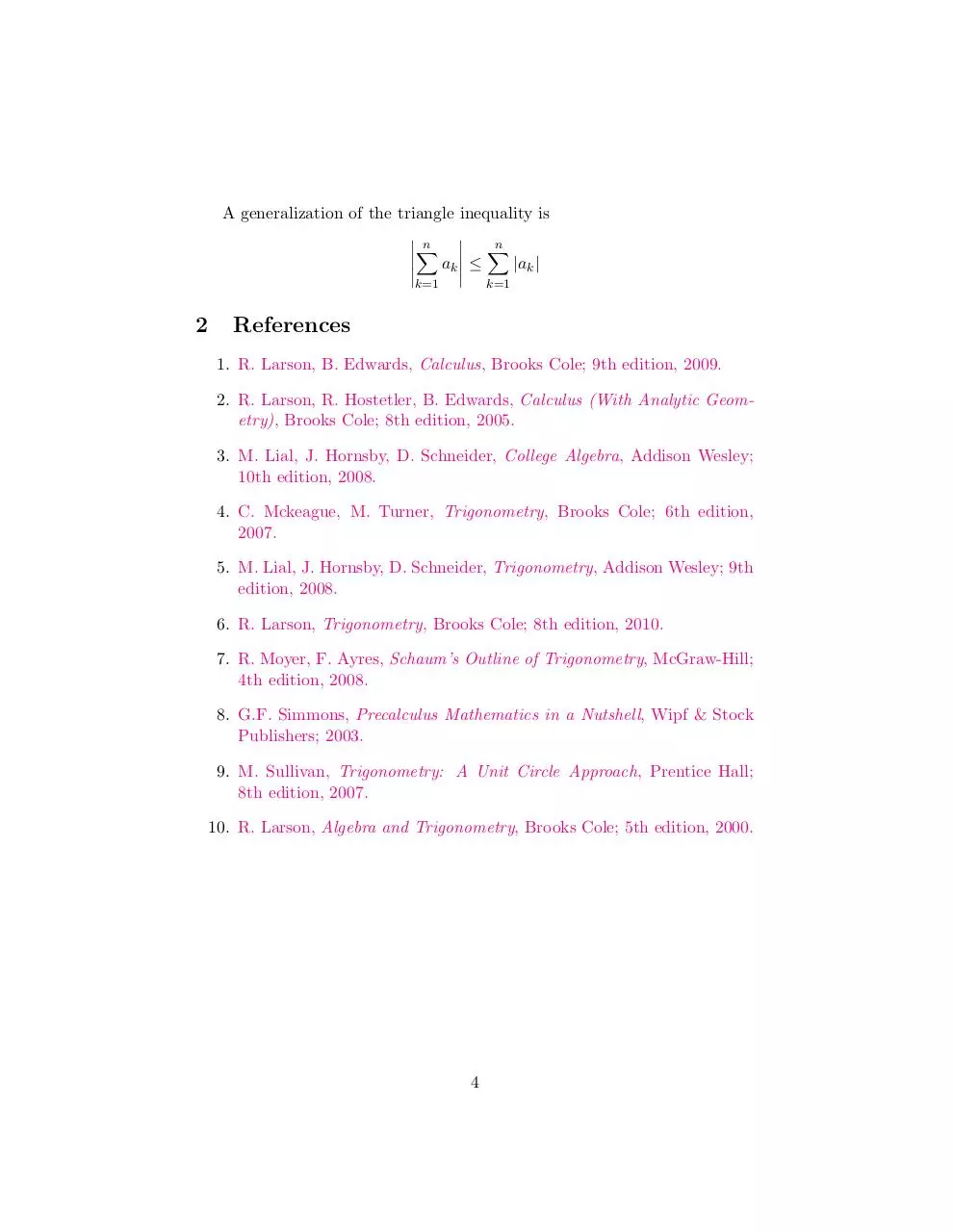# triangleineq .pdf

### File information

Title: 000.2 - The Triangle Inequality
Author: James Bonnar

This PDF 1.4 document has been generated by LaTeX with hyperref package / pdfTeX-1.40.3, and has been sent on pdf-archive.com on 28/07/2011 at 13:39, from IP address 98.144.x.x. The current document download page has been viewed 1805 times.
File size: 92.83 KB (4 pages).
Privacy: public file

### Document preview

000.2 - The Triangle Inequality
c
2010
Treasure Trove of Mathematics

The Cauchy-Schwarz inequality and the triangle inequality have many theoretical and practical applications including, but not limited to, normed
vector spaces, inner product spaces, p-norms and metric spaces.1 We shall
see that the Cauchy-Schwarz inequality is helpful in a proof of the triangle
inequality.
In its most basic form, the triangle inequality states that for any triangle,
the sum of the lengths of any two sides must be greater than the length of
the remaining side. In Euclidean geometry and some other geometries the
triangle inequality is a theorem about distances. For Euclidean right triangles it is a consequence of Pythagoras’ theorem, and for general Euclidean
triangles a consequence of the law of cosines, although it may optionally be
proven without these theorems.

1

Derivations and Proofs

Lemma. Suppose we have a triangle labeled by the vertex points O, A, and B,
sides a, b and a − b, and angle θ, as shown in Figure 1, and consider all
lengths and angles to be positive quantities. Then
a · b = ||a|| ||b|| cos θ
Proof of the Dot Product Formula. Given two vectors a = (a1 , a2 , a3 ) and
b = (b1 , b2 , b3 ), the dot product is defined as
a · b = a1 b1 + a2 b2 + a3 b3
Note that for any particular vector a, we have
a · a = a1 a1 + a2 a2 + a3 a3 = ||a||2
1

This document may be freely distributed, but not altered.

1

B
b−a
b
A
θ

a

O

Figure 1: The three vectors above form the triangle AOB and note that
the length of each side is nothing more than the magnitude of the vector
forming that side.
Now the law of cosines says,
||a − b||2 = ||a||2 + ||b||2 − 2 ||a|| ||b|| cos θ
We can write the left-hand side as,
||a − b||2 = (a − b) · (a − b)
= a·a−a·b−b·a+b·b
= ||a||2 − 2a · b + ||b||2
So we arrive at,
||a − b||2 = ||a||2 + ||b||2 − 2 ||a|| ||b|| cos θ
||a||2 − 2a · b + ||b||2 = ||a||2 + ||b||2 − 2 ||a|| ||b|| cos θ
−2a · b = −2 ||a|| ||b|| cos θ
a · b = ||a|| ||b|| cos θ

2

Theorem. (Cauchy-Schwarz Inequality). For any vectors u and v,
both in R2 or both in R3 ,
|u · v| ≤ ||u|| ||v|| .

(1)

The absolute value of the scalar product of two vectors is less than or equal
to the product of their norms.
Proof of the Cauchy-Schwarz Inequality. If u = 0 or v = 0, then (1) just
says 0 ≤ 0, which is true. Next suppose that u and v are nonzero vectors.
Then, as shown previously,
u · v = ||u|| ||v|| cos θ
where θ is the angle between the vectors. Taking absolute values and using
the fact that | cos θ| ≤ 1,
|u · v| = ||u|| ||v|| | cos θ| ≤ ||u|| ||v||

Theorem. (Triangle Inequality). For any vectors u and v, both in R2
or both in R3 ,
||u + v|| ≤ ||u|| + ||v|| .
(2)
The norm of the sum of two vectors is less than or equal to the sum of their
norms.
Proof of the Cauchy-Schwarz Inequality. Since both sides of (2) are nonnegative, (1) is equivalent to (meaning (1)and (2) imply each other):
(||u + v||)2 ≤ (||u|| + ||v||)2 .
So we only need to show this to complete the proof.
(||u + v||)2 = (u + v) · (u + v)
= u·u+u·v+v·u+v·v
= ||u||2 + 2u · v + ||v||2
≤ ||u||2 + 2|u · v| + ||v||2
(by Cauchy-Schwarz) ≤ ||u||2 + 2 ||u|| ||v|| + ||v||2
= (||u|| + ||v||)2

3

A generalization of the triangle inequality is
n

n
X
X

|ak |
ak ≤

k=1

k=1

2

References
1. R. Larson, B. Edwards, Calculus, Brooks Cole; 9th edition, 2009.
2. R. Larson, R. Hostetler, B. Edwards, Calculus (With Analytic Geometry), Brooks Cole; 8th edition, 2005.
3. M. Lial, J. Hornsby, D. Schneider, College Algebra, Addison Wesley;
10th edition, 2008.
4. C. Mckeague, M. Turner, Trigonometry, Brooks Cole; 6th edition,
2007.
5. M. Lial, J. Hornsby, D. Schneider, Trigonometry, Addison Wesley; 9th
edition, 2008.
6. R. Larson, Trigonometry, Brooks Cole; 8th edition, 2010.
7. R. Moyer, F. Ayres, Schaum’s Outline of Trigonometry, McGraw-Hill;
4th edition, 2008.
8. G.F. Simmons, Precalculus Mathematics in a Nutshell, Wipf &amp; Stock
Publishers; 2003.
9. M. Sullivan, Trigonometry: A Unit Circle Approach, Prentice Hall;
8th edition, 2007.

10. R. Larson, Algebra and Trigonometry, Brooks Cole; 5th edition, 2000.

4triangleineq.pdf (PDF, 92.83 KB)

### Share on social networks

#### HTML Code

Copy the following HTML code to share your document on a Website or Blog